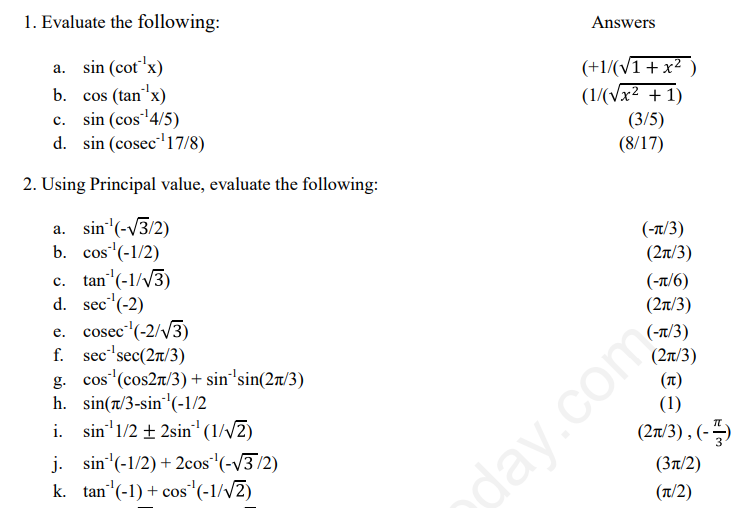# CBSE Class 12 Mathematics Inverse Trigonometric Functions Assignment Set B

Read and download free pdf of CBSE Class 12 Mathematics Inverse Trigonometric Functions Assignment Set B. Get printable school Assignments for Class 12 Inverse Trigonometric Functions. Standard 12 students should practise questions and answers given here for Inverse Trigonometric Functions in Grade 12 which will help them to strengthen their understanding of all important topics. Students should also download free pdf of Printable Worksheets for Class 12 Inverse Trigonometric Functions prepared as per the latest books and syllabus issued by NCERT, CBSE, KVS and do problems daily to score better marks in tests and examinations

## Inverse Trigonometric Functions Assignment for Class 12

Class 12 Inverse Trigonometric Functions students should refer to the following printable assignment in Pdf in standard 12. This test paper with questions and answers for Grade 12 Inverse Trigonometric Functions will be very useful for exams and help you to score good marks

### Class 12 Inverse Trigonometric Functions Assignment Pdf

CBSE Class 12 Mathematics Inverse Trigonometric Functions Assignment Set B. Chapter wise assignments are being given by teachers to students to make them understand the chapter concepts. Its extremely critical for all CBSE students to practice all assignments which will help them in gaining better marks in examinations. All assignments available for free download on the website are developed by the best teachers having many years of teaching experience in CBSE schools all over the country. Students, teachers and parents are advised to download the CBSE study material.Please click the below link to access CBSE Class 12 Mathematics Inverse Trigonometric Functions Assignment Set B

 CBSE Class 12 Mathematics Inverse Trigonometric Functions Assignment Set A CBSE Class 12 Mathematics Inverse Trigonometric Functions Assignment Set B CBSE Class 12 Mathematics Inverse Trigonometric Functions Assignment Set C CBSE Class 12 Mathematics Inverse Trigonometric Functions Assignment Set D CBSE Class 12 Mathematics Inverse Trigonometric Functions Assignment Set E CBSE Class 12 Mathematics Inverse Trigonometric Functions Assignment Set F CBSE Class 12 Mathematics Inverse Trigonometric Functions Assignment Set G